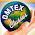Economics Board Paper October 2014 with solution

Economics Board Paper October 2014 with solution
Q1. A. Fill in the blanks with proper alternatives given in the brackets:

(1) Microeconomics is a study of ____________
[whole economy/ individual economic unit / general price level/ national output]
(2) The slope of demand curve is ___________ in the case of relatively inelastic demand.
[flatter/ steeper/ horizontal / vertical]
(3) The book ‘The General Theory of Employment, Interest and Money’ was written by __________
[Marshall/ Keynes / Smith/ Ricardo]
(4) Every loan creates a ___________
[loss/ profit / deposit/ credit]
(5) _____________ is an example of direct tax.
[Excise duty/ Income tax / Sales tax / Gifts]

(B) Match the following Group ‘A’ with Group ‘B’
 Group A Group B (1) Inferior goods (a) Average Cost (AC) (2) Reward of capital (b) 1st April to 31st March (3) Financial year (c) Quantitative measure of credit control (4) Bank rate (d) Marginal cost (5) Total Cost (TC) / Total Quantity (TQ) (e) 1st January to 31st December (f) Profit (g) Interest (h) Giffen goods

(C) State whether the following statements are true or false. (6)
(1) Demand for electricity is elastic. (TRUE)
(2) Supply is directly related to price. (TRUE)
(3) In monopolistic completion goods have no close substitutes. (FALSE)
(4) Barter system did not have any difficulty. (FALSE)
(5) D-mat account is useful to investors who deal in shares. (TRUE)
(6) Clearing house system economises the use of cash. (TRUE)

Q2. (A) Define or Explain the following  concepts (Any Three) (6)

(3) Monopoly
(4) Land

(B) Give reasons or Explain the following statements (Any Three) (6)

Q3. (A) Distinguish between the following. (Any Three) (6)

(B) Write short notes on (Any Two) (6)

Q4. Write short answers for the following questions. (Any Three) (12)

Q5. Explain, with reasons, whether you Agree or Disagree with the following statements. (Any Three) (12)

Q6. Write explanatory answers. (Any Two) 

Q6. Write explanatory answers. (Any Two):  (16)   Explain any ‘two methods’ of measuring price elasticity of demand. Explain the practical difficulties involved in the measurement of national income. State and explain the law of demand with assumptions. What is elasticity of demand? Explain the types of price elasticity of demand. Explain Keynesian psychological law of consumption. Explain in detail ‘saving function’ with schedule and diagram. What is ‘elasticity of demand’? Explain the factors determining elasticity of demand. What is ‘national income’ ? Explain the theoretical difficulties involved in the measurement of national income. Explain the various methods of measuring national income. What is aggregate supply? Explain the determinants of aggregate supply. State and explain in detail the Law of Diminishing Marginal Utility. Assumption to the Law of Diminishing Marginal Utility. Exception to the Law of Diminishing Marginal Utility. Define perfect competition and explain price determination under perfect competition. What is Aggregate demand? Explain the determinants of Aggregate demand. Exception to the Law of Demand What is perfect competition? Explain price determination under perfect competition. What is national income. Explain how national income is measured by output method. Explain Ratio method and Geometric method of measuring price elasticity of demand. Explain the subjective and objective factors determining consumption function.

1 comment:

1.hsc economics board paper october 2014.